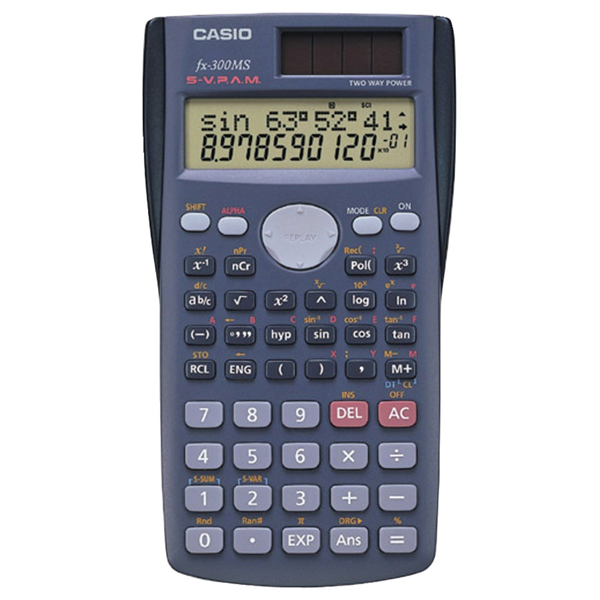# Casio fx-300MS Plus Scientific CalculatorSKU:
1073409

# Casio fx-300MS Plus Scientific Calculator

 \$15.95
Description:

### Casio FX-300MS Plus Scientific Calculator: Fast and Functional

• Casio Plus scientific calculator offers 229 in-built functions such as fraction/decimal conversions, trigonometry, fractions, regression analysis and more.
• Scientific calculator includes slide-on hard case and 2-line display for easy reading.
• Number of display digits: 10
• Solar plus means calculator has battery backup in dim light, auto power down feature
• Power source: Solar and battery
• Display notation: Numeric, display type: LCD
• Memory: 7K
• Dimensions: 3" x 5"
• Replacement batteries: LR44
• Protective cover: Hard case
• Display lines: 2
• Entry logic: Algebraic
• Percent key, +/- switch key, backspace key, square root key
• Levels of parentheses: 18
• Variable statistics: Two
• Fraction calculations, fraction/decimal conversions, decimal function, base number calculations, complex number calculations, hyperbolic functions, linear regression, logical (boolean) operations, polar rectangular conversion, probability, trig/log functions
• SKU:
1073409
Description:

### Casio FX-300MS Plus Scientific Calculator: Fast and Functional

• Casio Plus scientific calculator offers 229 in-built functions such as fraction/decimal conversions, trigonometry, fractions, regression analysis and more.
• Scientific calculator includes slide-on hard case and 2-line display for easy reading.
• Number of display digits: 10
• Solar plus means calculator has battery backup in dim light, auto power down feature
• Power source: Solar and battery
• Display notation: Numeric, display type: LCD
• Memory: 7K
• Dimensions: 3" x 5"
• Replacement batteries: LR44
• Protective cover: Hard case
• Display lines: 2
• Entry logic: Algebraic
• Percent key, +/- switch key, backspace key, square root key
• Levels of parentheses: 18
• Variable statistics: Two
• Fraction calculations, fraction/decimal conversions, decimal function, base number calculations, complex number calculations, hyperbolic functions, linear regression, logical (boolean) operations, polar rectangular conversion, probability, trig/log functions